Are you more of a visual learner? Check out our online video lectures and start your physics course now for free!Image: “Motion involves a change in position, such as in this perspective of rapidly leaving Yongsan Station.” No machine-readable author provided. License: CC BY 2.5

## Definition of Motion

### Uniform linear motion

Uniform linear motion is a motion that occurs at a constant speed in one direction. It means that an object covers equal displacements in equal intervals of time. In this case, acceleration is 0. This type of motion can be described in the following terms: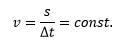v ⇒ velocity (m/s)
s ⇒ displacement (m)
t ⇒ time (s)

### Motion with uniform acceleration

This type of motion can be characterized by the changing velocity. The object is moving faster or slower, which means that acceleration does not equal 0 and remains constant. Motion with uniform acceleration can be described by 3 equations:

Displacement-time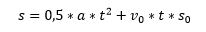Velocity-time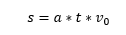s (or s0) ⇒ displacement (m)

a ⇒ acceleration (m/s2)

t ⇒ time (s)

v0 ⇒ velocity (m/s)

### Free fall

Free fall is the motion of an object where the force of gravity is the only force acting upon it. The force of gravity is a constant parameter with an acceleration a = g = 9.81 m/s. Thus, free fall refers to motion with uniform acceleration. After neglecting air friction and lift, the following equations remain: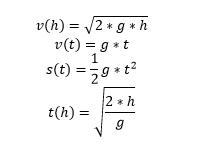g = 9.81 m/s

h ⇒ height

### Uniform circular motion

In this type of motion, an object is moving along a circular path. Since the velocity is a vector, its constantly changing directions balance each other out. Thus, uniform circular motion is defined by the constant sum of velocity or to put it simply, if a car is driving in a circle with the speed of 50 km/h, the acceleration is constant, yet the direction constantly changes.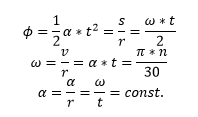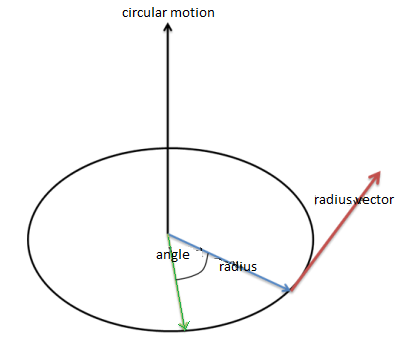ω ⇒ angular velocity (1/s)
α ⇒ angular acceleration (1/s2)
n ⇒ rotational speed (1/s)
π ⇒ Pi (approx. 3.14)

### Periodic motion

The periodic motion refers to changes in a system or a physical variable based on a fixed position that is repeated approx. or exactly in equal intervals of time. It can be described with the following variables: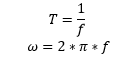T ⇒ period, measured in seconds (s)
f ⇒ frequency, measured in hertz (Hz)
ω ⇒ angular velocity (1/s)

## Graphs of Motion

The types of motion described above can be represented in the following graphs. Uniform linear motion is displayed in red, and motion with uniform acceleration is displayed in green.

Displacement-time graph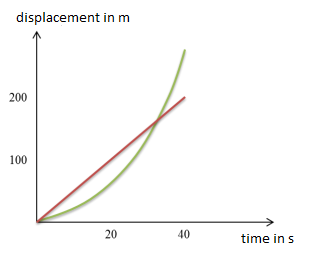Velocity-time graph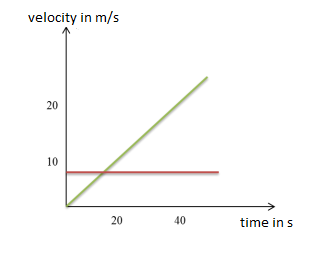Acceleration-time graph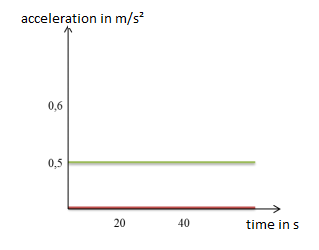## Visualizing Kinematics

### Definition

Kinematics is associated with the geometry of motion, and thus, it is a branch of mathematics. It begins with a description of the geometry of the system with the initial conditions of known values. These values depict the position, the velocity, and/or the acceleration of various points that are part of the system. Kinematics helps describe the motion of points, bodies, and groups of bodies without taking into account the masses of the objects or the forces that cause the motion.

### Visualization aspect

Kinematics can be displayed using two-dimensional graphs. The plots would help understand the motion of the object with respect to time. Usually, 3 graphs are utilized: position graph, velocity graph, and acceleration graph.

The position graph displays the location of the object as time passes.
The velocity graph displays the speed of the object as time passes.
The acceleration graph displays how fast the object is moving as time passes.

The graph is two-dimensional, so it contains an x-axis and a y-axis. The x-axis represents time in seconds. The y-axis can represent any of the 3 items aboveposition (also called displacement), velocity, and acceleration.

## Vectors

### Definition

Vector is a quantity possessing both magnitude and direction. This quantity is different from a scalar quantity that has magnitude but no direction. For instance, if one checks the temperature, the result is a scalar quantity since temperature does not have direction. However, if one measures the wind, it is a vector quantity. The wind has both magnitude and direction since 30 mph from the west implies that the magnitude is 30 mph, and the direction is from the west.

## Projectile Motion

Projectile motion is a form of motion in which an object (referred to as the projectile) is thrown near the earth’s surface moving along a curved path under the action of gravity only. In this case, air resistance is treated as being negligible. The path the object takes is parabolic, with the only force acting on it being gravity (acceleration), which was stated before as equal to 9.8 m/sec2.

## Components of Projectile Motion

The components in the x-axis and y-axis are calculated differently.

In the horizontal axis (x-axis), acceleration is 0 since neither wind nor air resistance is present. The velocity in the horizontal axis will equal the initial velocity in the horizontal axis. The distance traveled in the horizontal axis (displacement) can also be determined based on the time traveled. The following equations can be used:

ax = 0

(ax = acceleration in x-direction)

vx = v0x

(vx = velocity in x-direction, v0x = original velocity in x-direction)

x = x0 + v0x t

(x = displacement in x-direction, x= initial position, t = time)

In the vertical axis (y-axis), acceleration is equal to gravity. The velocity and displacement equations will be different since acceleration will affect the object in the vertical axis.

ay = -g

(ay = acceleration in y-direction, g = gravity)

vy = voy – g t

(vy = velocity in y-direction, voy = original velocity in y-direction, t = time)

y = yo + voy t – ½ g t2

(y = displacement in y-direction, y0 = initial position)

## List of Parameters

 t (or t0) Time (s) v (or v0) Velocity (m/s) s (or s0) Displacement (m) a Acceleration (m/s2) g = 9.81 m/s2 Acceleration of gravity (m/s2) h Height (or falling height) α Angular acceleration s Arc length or displacement (m) r Radius (m) n Rotational speed (1/s) v Velocity (m/s) T Period, measured in seconds (s) f Frequency, measured in hertz (Hz) ω Angular velocity (the frequency of circular motion per second) (1/s) Φ Exceeded angle p Momentum (kg m/s or N s) m Mass (kg) F Force (N or kg m/s2) I → Impulse (kg m/s) Fav Average or net force (N or kg m/s2) Ms Center of mass, no unit M Torque (N m) J Moment of inertia (kg m2) r Axis of rotation ρ Mass distribution L Angular momentum [(kg m2)/s] p Angular momentum of point-mass [(kg m)/s] W Work (J or N m) E Energy (J or N m) P Power (W or J/s) Δp Collision [(kg m)/s] ρ Pressure (Pa or N/m2) A Area of a surface (m2) V Volume (m3 or L)

Learn. Apply. Retain.
Your path to achieve medical excellence.
Study for medical school and boards with Lecturio.(Votes: 7, average: 4.29)Loading...